Ch 12. Infinite Sequences and Series Multimedia Engineering Math Sequencesand Series Integral andComparison Alt. Series &Abs. Conv. PowerSeries Taylor, Mac. &Binomial
 Chapter 1. Limits 2. Derivatives I 3. Derivatives II 4. Mean Value 5. Curve Sketching 6. Integrals 7. Inverse Functions 8. Integration Tech. 9. Integrate App. 10. Parametric Eqs. 11. Polar Coord. 12. Series Appendix Basic Math Units Search eBooks Dynamics Fluids Math Mechanics Statics Thermodynamics Author(s): Hengzhong Wen Chean Chin Ngo Meirong Huang Kurt Gramoll ©Kurt GramollMATHEMATICS - CASE STUDY SOLUTION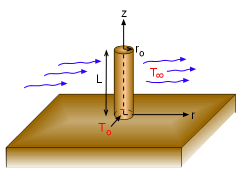A Circular Fin Protruding from a
Hot Wall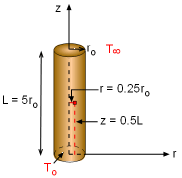Point of Interest: r/ro = 0.25
and z/L = 0.5

It is given that the two-dimensional steady-state temperature distribution of a circular fin is: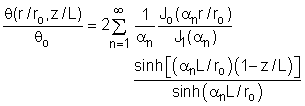For r/ro = 0.25, L/ro = 5, and z/L = 0.5, the above equation reduces to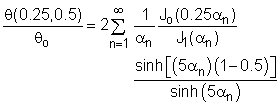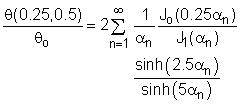where the first four zeros of Jon) are given as:
α1 = 2.405, α2 = 5.520, α3 = 8.654, and α4 = 11.792

The next step is to determine the Bessel functions using five terms of the power series expansion: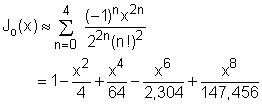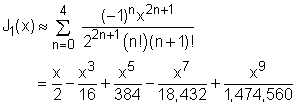For α1 = 2.405, α2 = 5.520, α3 = 8.654, and α 4 = 11.792, the Bessel functions and hyperbolic sine functions are summarized in the following table:

 n αn Jo(αn/4) J1(αn) sinh(2.5αn) sinh(5αn) 1 2.405 0.9116 0.5192 204.3 8.34×104 2 5.520 0.5777 0.3487 4.92×105 4.85×1011 3 8.654 0.1309 77.63 1.24×109 3.10×1018 4 11.792 -0.2388 1,767 3.18×1012 2.02×1025

The dimensionless temperature at r/ro = 0.25, L/ro = 5, and z/L = 0.5 becomes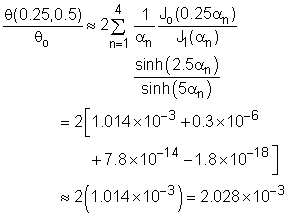Is the approximation for the Bessel functions good enough by only including five terms of the power series expansion? Use the simulation in this section to check the accuracy. Suppose that the first four zeros of Jo(α) are not given in the assumption, at least how many terms in the power series expansion do you need in order to obtain a reasonable solution?